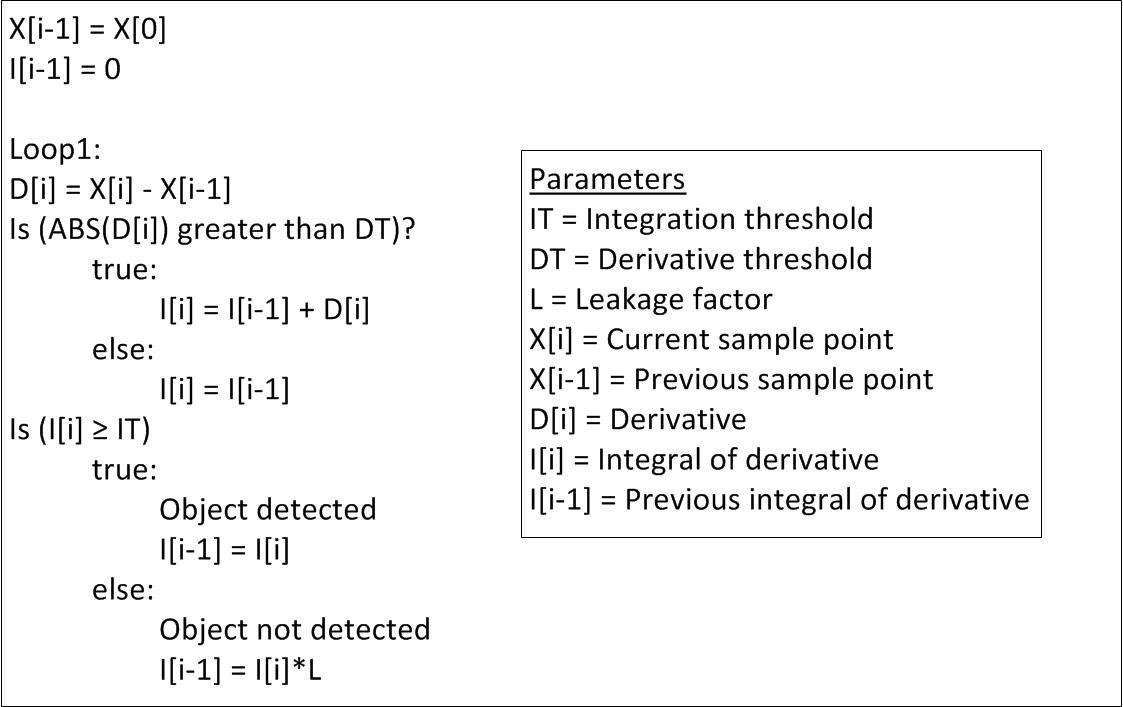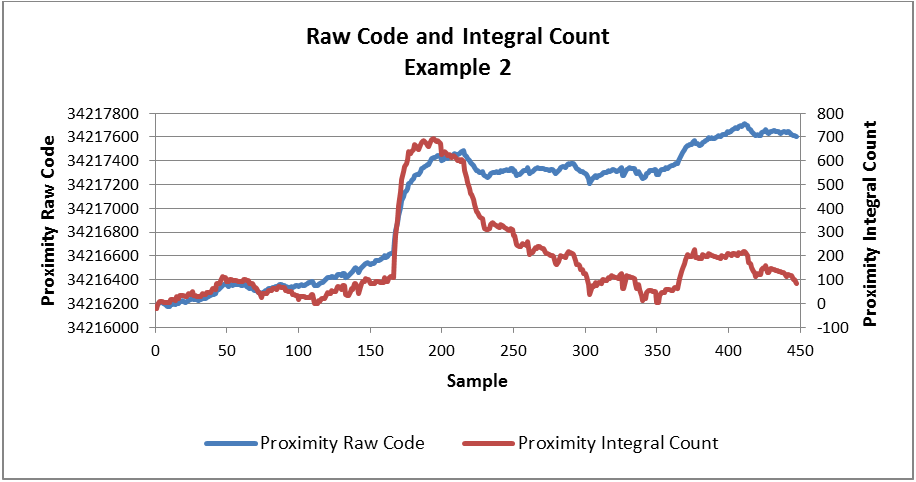# Capacitive sensing: simple algorithm for proximity sensing

Proximity-sensing applications require the detection of small changes in capacitance (typically on the order of a few femtofarads) around the noise floor. Since there are many ways to process the data to determine whether a target was detected or not, how do you choose the best method? In this post, I’ll describe a simple algorithm that you can use for proximity sensing or capacitive touch applications that does not require significant processing overhead.

Derivative integration algorithm

A derivative integration algorithm (see the pseudo code shown in Figure 1) is a simple way to process the data. You can use it for both proximity sensing and capacitive touch buttons; the only difference between the two would be the derivative and integration thresholds for each sensor to obtain a robust and highly sensitive response.Figure 1: Pseudo code for the derivative integration algorithm

This algorithm tracks the rate of change or derivative, D[i], between the current measurement, X[i], and the previous measurement, X[i-1]. Proximity-sensing applications require the detection of small capacitance changes. This requires the derivative threshold, DT, to be very low. Changes in capacitance due to noise can be a severe problem, especially if the DT is very low. The integral of the derivative, I[i], can start to accumulate and falsely trigger as a detected object. Random noise should stabilize the integral value so that the mean is zero (no capacitance drift occurs), but a high integration threshold, IT, can allow enough noise margin for nonrandom noise.

The leakage factor, L, is a value between 0 (instant dissipation) and 1 (no dissipation). It is typically set at 0.99 to represent that the algorithm has some memory of past values to determine where the detection boundary occurs. You can use various leakage factors for a faster recovery time if the integral swings too far positive. This will cause temporary sensitivity reduction until the integral can stabilize near 0.

Once a human hand approaches the sensing area, the integration value will start to accumulate as long as the derivative of the measurements hits the derivative threshold. If the device detects the hand (the integral value goes below the integration threshold) and the hand stops in the sensing area, the derivative flattens out and the integral stops accumulating. As the hand moves away from the sensor, the integral recedes until it goes above the integral threshold. This indicates that the target object is outside the intended sensing range.

For capacitive touch applications, a low derivative threshold is not required. You can optimize the integration threshold based on the desired button response. You can also implement multiple derivative and integration thresholds to filter out any high-frequency noise seen in the sampled measurements and increase the sensitivity response.

Figure 2 shows an example of a waveform with a target detected using the FDC2214. As the derivative becomes more negative (an object approaches the proximity sensor), the integral count matches the raw code signal, as expected. You can also optimize the thresholds to be robust against any slow-moving drift. Figure 3 shows how a drift in the raw code is compensated in the integral count. The signal is preserved without any distortions due to the slow upward drift.

Figure 2: Example 1 – proximity raw code, derivative and integral waveformsFigure 3: Example 2 – raw code and integral count

IIR filter implementation

To improve the signal-to-noise ratio in the measurements, you can implement an Infinite Impulse Response (IIR) filter to process the data and obtain an average measurement before sending it through the derivative integration algorithm. The IIR filter implementation is similar to a moving average except that previous values are not stored and shifted out of the summing order. This process saves memory and computational time at the expense of slight accuracy degradation.

The filter processes a running total of ‘N’ values. The current average (Avg[i]) is calculated by taking the previous average (Avg[i-1]), multiplying by N and subtracting the previous average. Then, the new value (val[i]) is added to the sum to create a “new sum”. Dividing by N creates a new average. Having N be multiples of two allows bit shifting instead of actually dividing, thus saving computational cycles.

Summary

The derivative integration algorithm is a simple algorithm that can be used to for proximity sensing and capacitive touch applications. This solution is a foundation that other sophisticated processing algorithms can be combined with to make the algorithm more robust for various system conditions. Depending on the system requirements, you will need to optimize the processing, but the derivation integration algorithm is a great start for quick prototyping.